Urban Damage Assessment from High Resolution Images using OSM MASK and Neural Network

A complete methodology has been given by Dariia Gordiiuk of Earth Observatory Systems(EOS) Data Analytics for detecting urban damages Applying Neural Network and Local Laplace Filter and OSM masking Methods to Very High Resolution Satellite Imagery. Hope it will help a lot the geospatial analysts.

Since the beginning of the human species, we have been at the whim of Mother Nature. Her awesome power can destroy vast areas and cause chaos for the inhabitants. The use of satellite data to monitor the Earths surface is becoming more and more essential. Of particular importance are the disasters and hurricane monitoring systems that can help people to identify damage in remote areas, measure the consequences of the events, and estimate the overall damage to a given area. From a computing perspective, such an important task needs to be implemented to assist in various situations.

To analyze and estimate the effects of a disaster, we use high-resolution, satellite imagery from an area of interest. This can be obtained from Google Earth. We can also get free OSM vector data that has a detailed ground truth mask of houses. This is the latest vector zip from New York (Figure 1).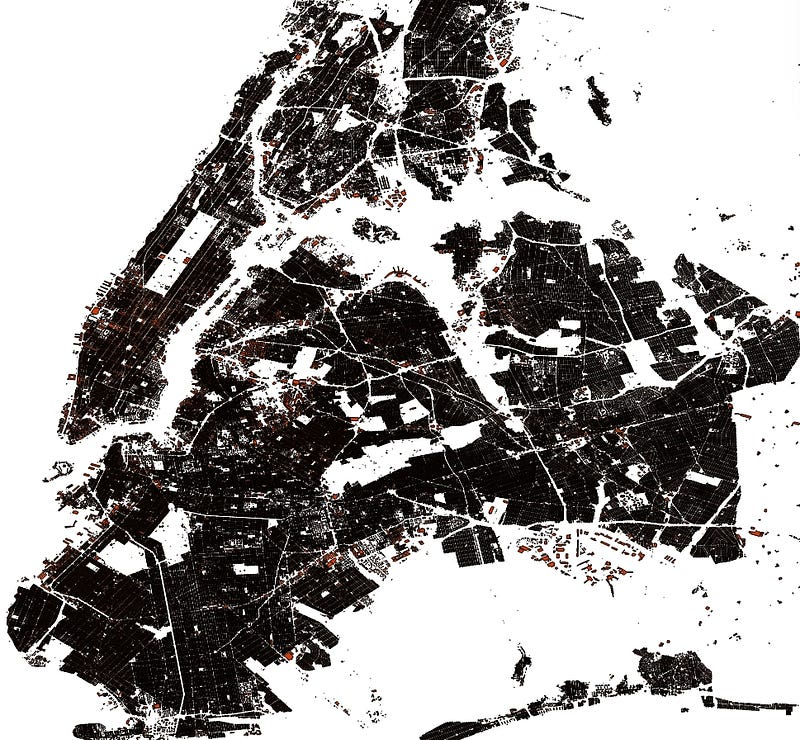Figure 1. NY Buildings Vector Layer

Next, we rasterize (convert from vector to raster) the image using a tool from gdal, called gdal_rasterize. As a result we have acquired a training and testing dataset from Long Island (Figure 2).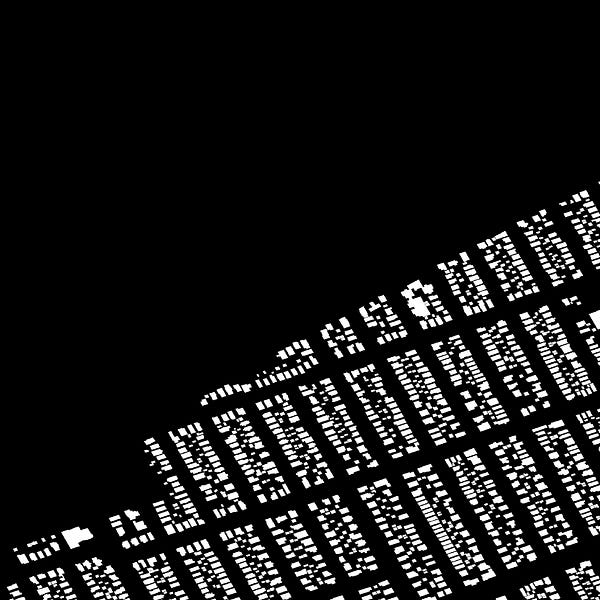Figure 2. Training Data Fragment of CNN

We apply a deep learning framework Caffe for training purposes and the learning model of Convolutional Neural Networks (CNN):

The derived neural net enables us to identify the predicted houses from the target area after the event (Figure 4). We can also use data from another similar area which hasn’t been damaged for CNN learning (if we can’t access the data for the desired territory).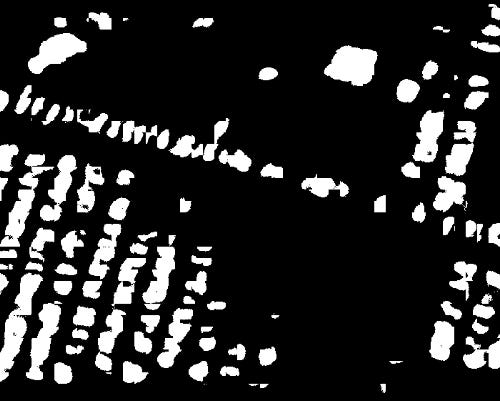Figure 4. Predictive Results of CNN Learning

We work with predicates of buildings using vectorization (extracting a contour and then converting lines to polygons) (Figure 5).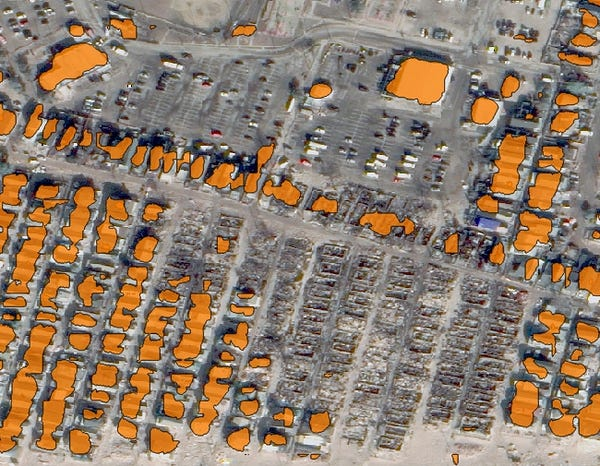Figure 5. Predictive Results of Buildings (Based on CNN)

Also, we need to compute the intersection of the obtained predicate vector and the original OSM vector (Figure 6). This task can be accomplished by creating a new filter, dividing the square of the predicate buildings by the original OSM vector. Then, we filter the predictive houses by applying a threshold of 10%. This means that if the area of houses in green (Figure 6) is 10% less than the area in red, the real buildings have been destroyed.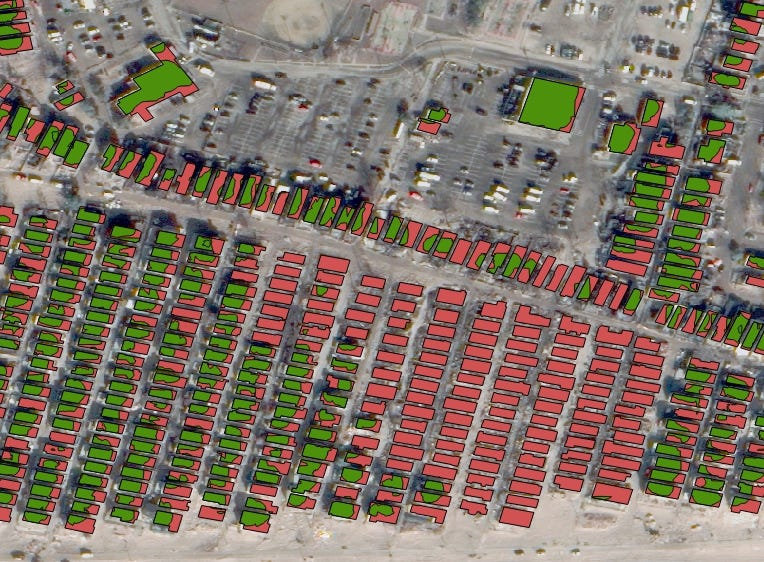Figure 6. Calculating CNN-Obtained Building Number (Green) Among Buildings Before Disaster (Red)

Using the 10%-area threshold we can remove the houses that have been destroyed and get a new map that displays existing buildings (Figure 7). By computing the difference between the pre- and post- disaster masks, we obtain a map of the destroyed buildings (Figure 8).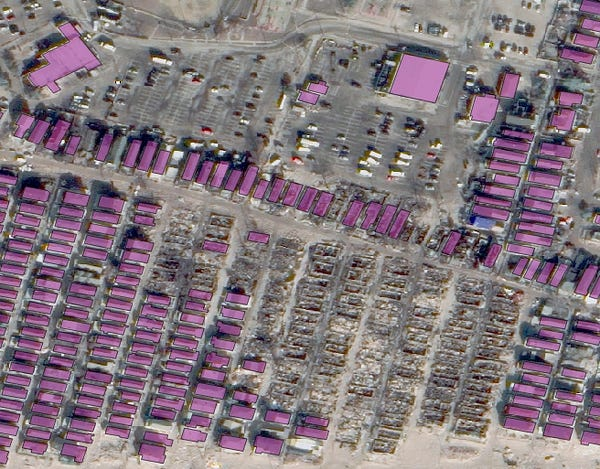Figure 7. Buildings: Before and After Disaster With CNN Method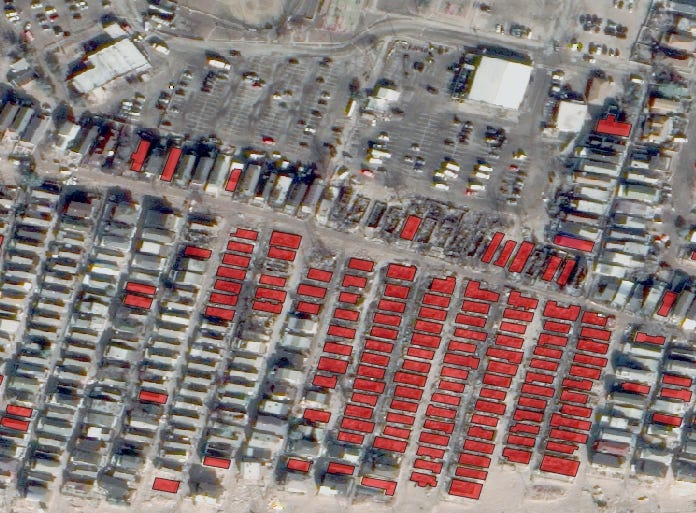Figure 8. Destroyed Buildings With CNN

We have to remember that the roofs of the houses are represented as flat structures in 2D-images. This is an important feature that can also be used to filter input images. A local Laplace filter is a great tool for classifying flat and rough surfaces (Figure 9). The first image has to be a 4-channel image with the fourth Alpha-channel that describes no-data-value pixels in the input image. The second image (img1) is the same, a 3-channel RGB image.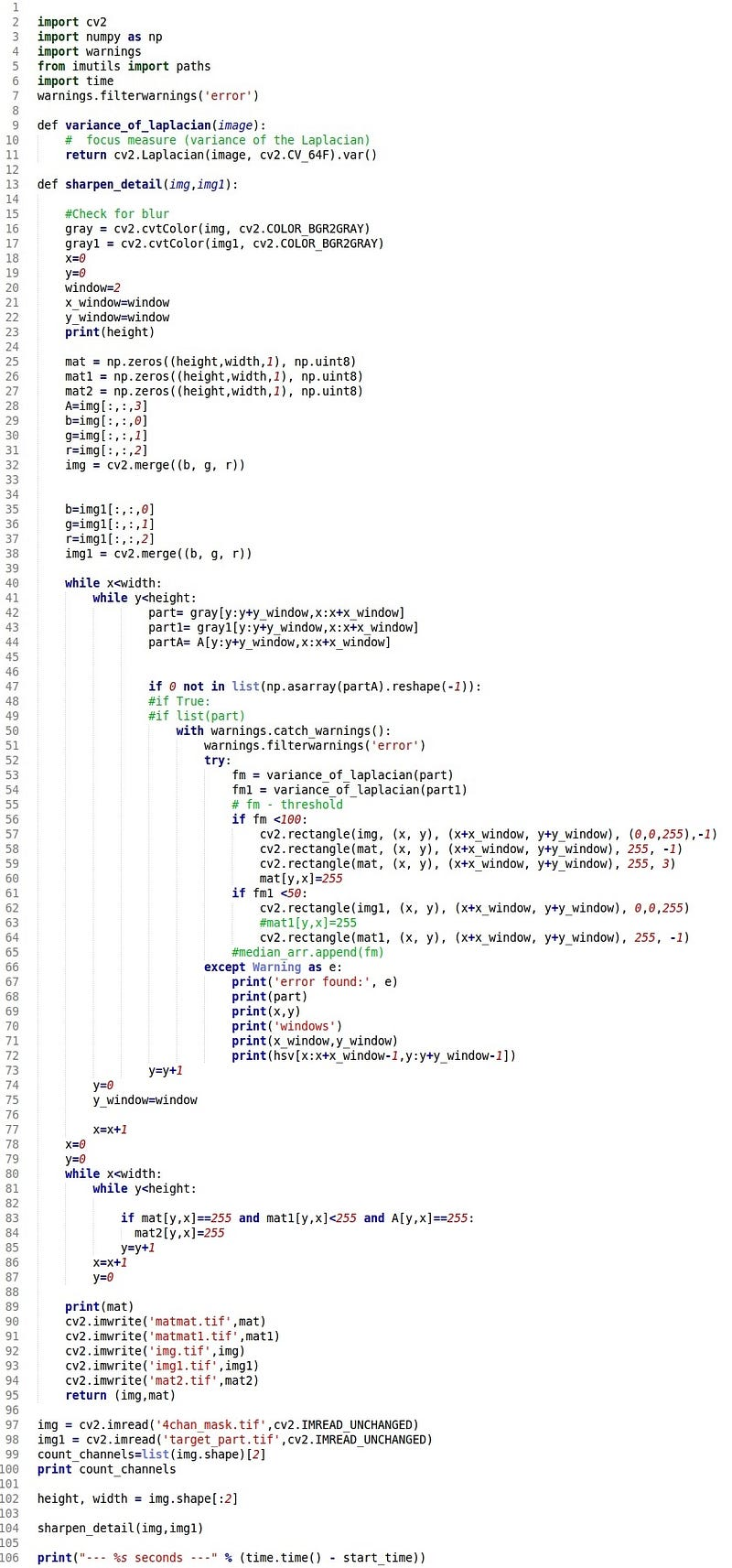Figure 9. Local Laplace Window Filter

Applying this tool lets you get the map of the flat surface. Let’s look at the new mask of the buildings which have flat and rough textures (Figure 10) after combining this filter and extracting the vector map.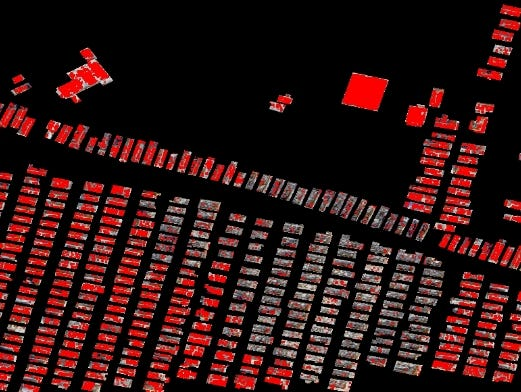Figure 10. Flat Surface Mask With Laplace Window Filter Followed By Extracted House Mask

A robust library of the OpenCV computer vision has a denoising filter that helps remove noise from the flat buildings masks (Figure 11, 12).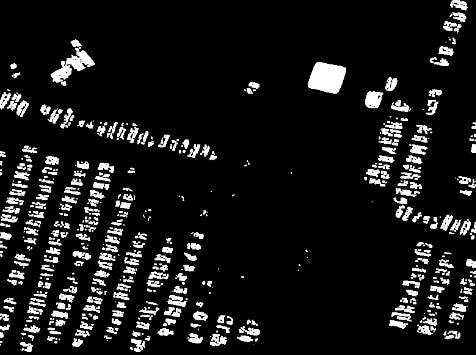Figure 12. Resulting Mask. Pre- and Post- Disaster Images After Applying Denoising Filter

Next, we apply filters to extract the contours and convert the lines into the polygons. This enables us to get new building recognition results (Figure 13).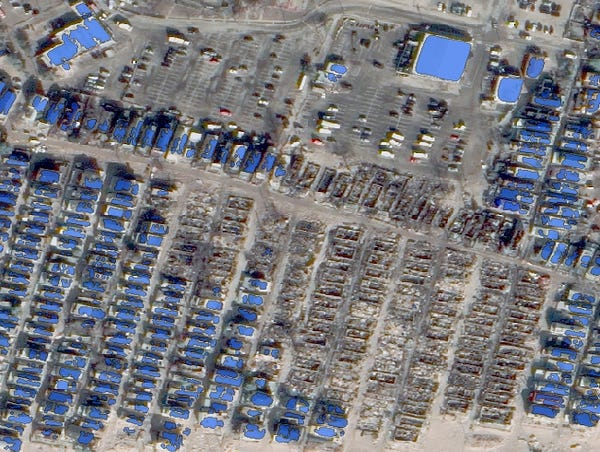Figure 13. Predictive Results of Buildings With Laplace Filter

We compute the area of an intersection vector mask obtained from the filter and a ground truth OSM mask and use a 14% threshold to reduce false positives (Figure 14).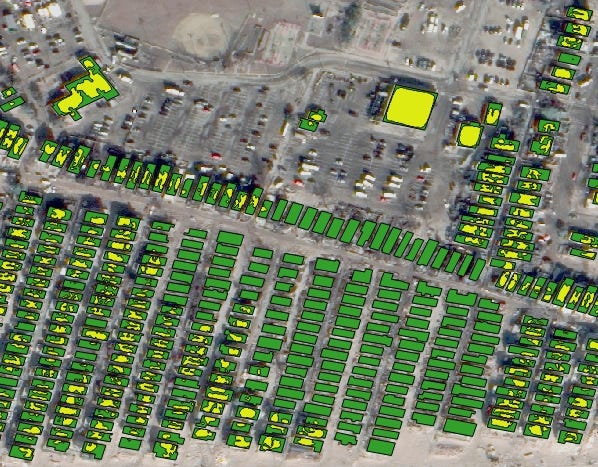Figure 14. Calculations: Buildings With Laplace Filter (Yellow) Before Damage (Green), Using 14% Threshold

As a result, we can see a very impressive new mask that describes houses that have survived the hurricane (Figure 15) and a vector of the ruined buildings (Figure 16).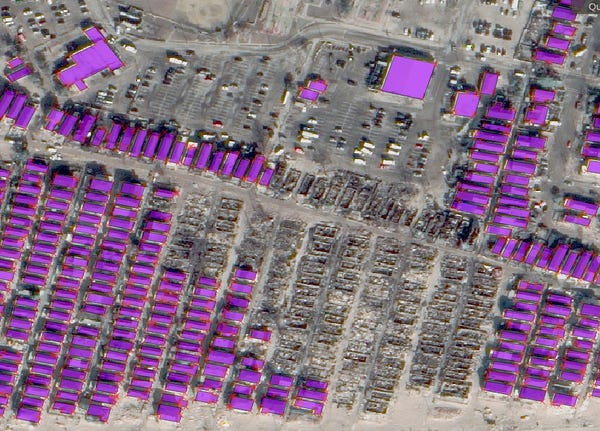Figure 15. Before and After Disaster With Laplace FilterFigure 16. Destroyed Buildings With Laplace Filter

After we have found the ruined houses, we can also pinpoint their location. For this task OpenStreetMap comes in handy. We have installed an OSM plugin in QGis and added an OSM layer to the canvas (Figure 17). Then, we added a layer with the destroyed houses and we can see all their addresses. If we want to get a file with the full addresses of the destroyed buildings we have to:

1. In QGis use Vector / OpenStreetMap / Download the data and select the images with the desired information.
2. Then in QGis use Vector / OpenStreetMap / Import a topology from XML and generate a DataBase from the area of interest.
3. QGis / Vector / Export the topology to Spatialite and select all the required attributes. (Figure 18)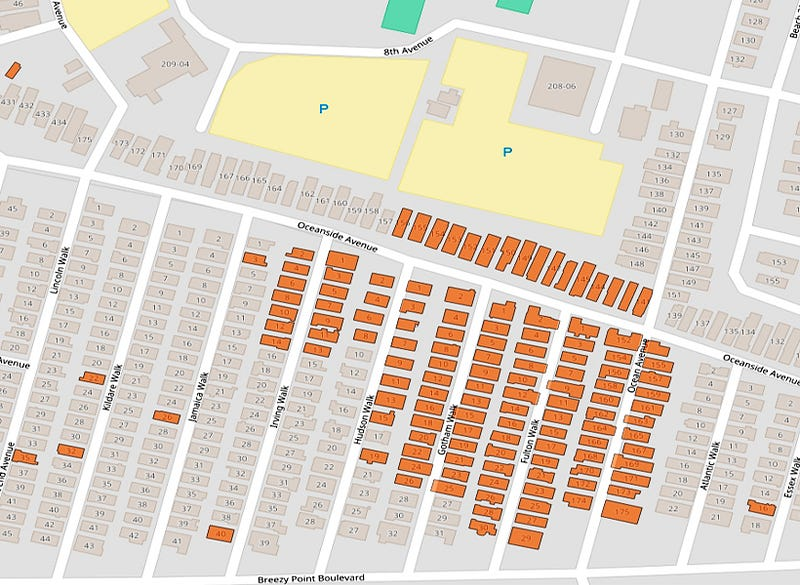Figure 17. Destroyed Houses Location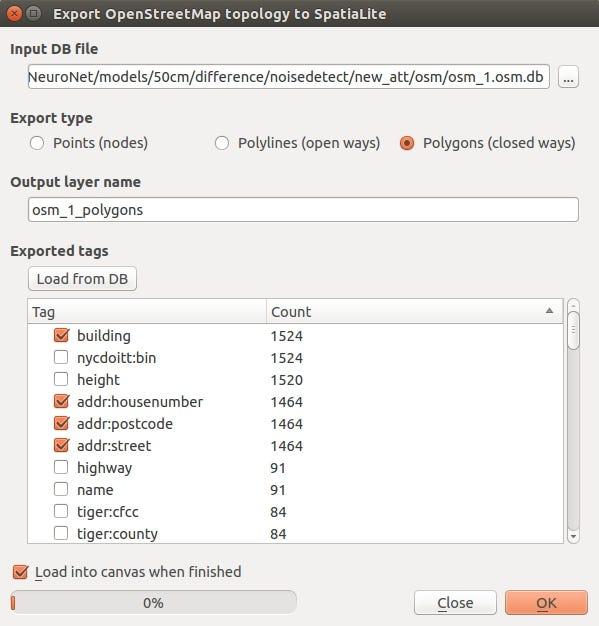Figure 18. Required Attributes Selection To Load Vector Into Ruined Buildings

As a result, we can get a full list, with addresses, of the destroyed buildings (Figure 19).